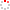CIOMP OpenIR  > 中科院长春光机所知识产出
 Design of super-elliptical gradient coils based on multiple objective Pareto optimization method Pan, H.; L. Wang; Q. L. Wang; L. M. Chen; F. Jia and Z. Y. Liu 2017 发表期刊 Acta Physica Sinica卷号 66期号:9 摘要 The design of gradient coils for a magnetic resonance imaging (MRI) system is a multiple objective optimization problem, which usually needs to deal with a couple of conflicting design objectives, such as the stored magnetic energy, power consumption, and target linear gradient distribution. These design requirements usually conflict with each other, and there is no unique optimal solution which is capable of minimizing all objectives simultaneously. Therefore, the design of gradient coils needs to be optimized reasonably with the tradeoff among different design objectives. Based on the developable property of the super-elliptical cylindrical surface and the stream function design method, the multiple objective optimization problem is analyzed by using the Pareto optimization method in this paper. The effect of proposed approach is illustrated by using the stream function method and three aforementioned coil design objectives are analyzed. The influences of the stored magnetic energy and power consumption target on linearity of gradient coil and the configuration of coils are analyzed respectively. The suitable sizes of gradient coils are discussed by analyzing the change of the stored magnetic energy. A weighted sum method is employed to produce the optimal Pareto solutions, in which the multiple objective problem reduces into a single objective function through a weighted sum of all objectives. The quantitative relationship of each design requirement is analyzed in the Pareto solution space, where Pareto optimal solutions can be intuitively found by dealing efficiently with the tradeoff among different coil properties. Numerical examples of super-elliptical gradient coil solutions are provided to demonstrate the effectiveness and versatility of the proposed method to design super-elliptical gradient coils with different coil requirements. The optimization results show that there are multiple available solutions in the convex Pareto solution space under the constraints that the linear gradient deviation is less than 5% and the magnetic stored energy and power dissipated are both no more than user-preset values. In the case that the values of summed objective functions are the same, the proposed method can intuitively see the performance of each individual target, thereby conducting to realizing the final design of gradient coils under the different design requirements. With the proposed approach, coil designers can have a reasonable overview of gradient coil design about the achievable performances of some specific properties and the competing or compatible relationships among coils properties. Therefore, a suitable design of the gradient coils for a given requirement of MRI application can be chosen reasonably. 收录类别 sci ; ei 语种 中文 文献类型 期刊论文 条目标识符 http://ir.ciomp.ac.cn/handle/181722/59148 专题 中科院长春光机所知识产出 推荐引用方式GB/T 7714 Pan, H.,L. Wang,Q. L. Wang,et al. Design of super-elliptical gradient coils based on multiple objective Pareto optimization method[J]. Acta Physica Sinica,2017,66(9). APA Pan, H.,L. Wang,Q. L. Wang,L. M. Chen,&F. Jia and Z. Y. Liu.(2017).Design of super-elliptical gradient coils based on multiple objective Pareto optimization method.Acta Physica Sinica,66(9). MLA Pan, H.,et al."Design of super-elliptical gradient coils based on multiple objective Pareto optimization method".Acta Physica Sinica 66.9(2017).
 条目包含的文件 下载所有文件 文件名称/大小 文献类型 版本类型 开放类型 使用许可 Design of.pdf（1326KB） 期刊论文 作者接受稿 开放获取 CC BY-NC-SA 浏览 下载
 个性服务 推荐该条目 保存到收藏夹 查看访问统计 导出为Endnote文件 谷歌学术 谷歌学术中相似的文章 [Pan, H.]的文章 [L. Wang]的文章 [Q. L. Wang]的文章 百度学术 百度学术中相似的文章 [Pan, H.]的文章 [L. Wang]的文章 [Q. L. Wang]的文章 必应学术 必应学术中相似的文章 [Pan, H.]的文章 [L. Wang]的文章 [Q. L. Wang]的文章 相关权益政策 暂无数据 收藏/分享
 文件名： Design of.pdf 格式： Adobe PDF